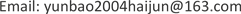AEAdvances in Education2160-729XScientific Research Publishing10.12677/AE.2019.92027AE-29171AE20190200000_95837858.pdf人文社科 液体粘滞系数测量实验中的几点思考 Suggestions on the Measurement of Liquid Viscosity Coefficient 21景峰21志浩2121禹权21内蒙古工业大学理学院物理学系，内蒙古 呼和浩特null130220190902133136© Copyright 2014 by authors and Scientific Research Publishing Inc. 2014This work is licensed under the Creative Commons Attribution International License (CC BY). http://creativecommons.org/licenses/by/4.0/Copyright © 2019 by author(s) and Hans Publishers Inc.1. 引言

2. 逐差法的应用

Q = d V d t = ∫ 0 a 2 π r v d r = π p a 4 8 η L (1)

t = ∫ 0 t d t = ∫ 0 t d V π a 4 p 8 η L = − 8 η L π ρ g a 4 ∫ y 1 y 2 d V y (2)

y1，y2分别为液体在不同时刻的高度，由此利用液体密度以及液体从y1到y2下落的时间t通过积分运算得到该液体的粘滞系数η。

t = ∫ 0 t d t = ∫ 0 t d V π a 4 p 8 η L = − 8 η L π ρ g a 4 ∫ y 1 y 2 d V y = − 8 η L π ρ g a 4 ∫ y 1 y 2 A d y y = − 8 η L A π ρ g a 4 ln y 2 y 1 (3)

η = − π ρ g a 4 t 8 A L ( ln y 2 − ln y 1 ) (4)

y 2 = y 1 e − b t (5)

ln y = ln ( y 1 e − b t ) = ln y 1 − b t (6)

b = − ∑ i = 1 5 ( ln h i + 5 − ln h i ) ∑ i = 1 5 ( t i + 5 − t i ) = ln ( ∏ i = 1 5 h i ∏ j = 6 10 h j ) ∑ i = 1 5 ( t i + 5 − t i ) (7)

y 2 = y 1 e − b t = y 1 ( 1 − b t ) (8)

b = − ∑ i = 1 5 ( y i + 5 − y i ) y 0 ∑ i = 1 5 ( t i + 5 − t i ) (9)

3. 起始测量高度对实验结果的影响

The relative error of the viscosity coefficient, the average velocity and the corresponding Reynolds number at different timing height

4. 曲线拟合与理论计算的比较